# Python | Hatching Head of the Arrow

In this article, we are going to hatch the head of the arrow (with default shape) using the hatch command of matplotlib.
Submitted by Anuj Singh, on July 31, 2020

Through this tutorial, we are trying to present different types of hatch that are possible in an arrow. Any of them can be used for different applications. Following are the plots as an output from the python code.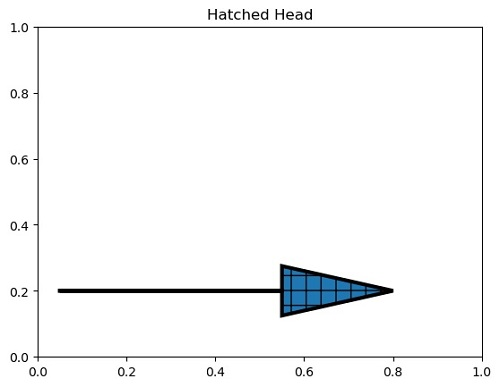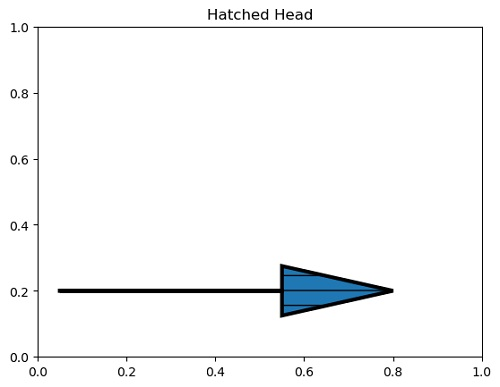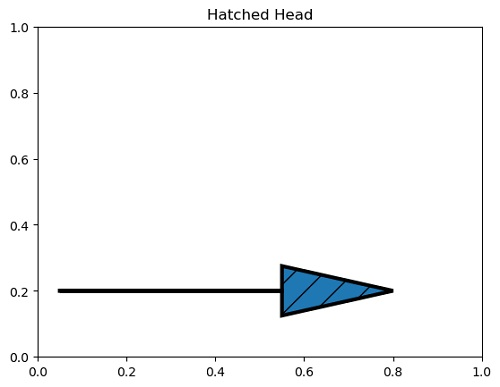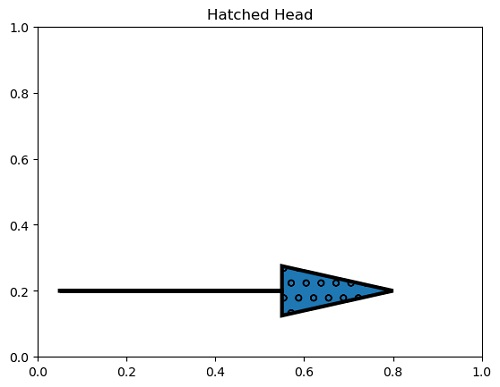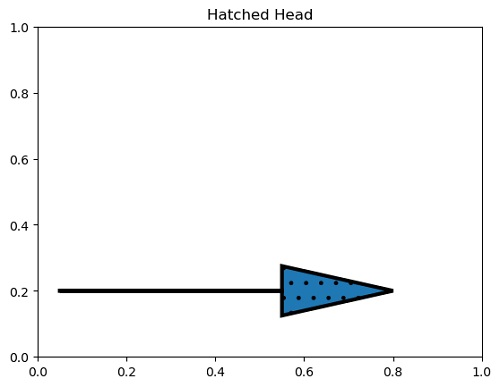## Python code for hatching head of the arrow

```import matplotlib.pyplot as plt

plt.figure()
plt.arrow(0.05, 0.2, 0.5, 0, linewidth=3.0, hatch='+',
plt.show()

plt.figure()
plt.arrow(0.05, 0.2, 0.5, 0, linewidth=3.0, hatch='-',
plt.show()

plt.figure()
plt.arrow(0.05, 0.2, 0.5, 0, linewidth=3.0, hatch='/',
plt.show()

plt.figure()
plt.arrow(0.05, 0.2, 0.5, 0, linewidth=3.0, hatch='o',
plt.show()

plt.figure()
plt.arrow(0.05, 0.2, 0.5, 0, linewidth=3.0, hatch='.',
plt.show()
```

Output:

```Output is as figure
```

Languages: » C » C++ » C++ STL » Java » Data Structure » C#.Net » Android » Kotlin » SQL
Web Technologies: » PHP » Python » JavaScript » CSS » Ajax » Node.js » Web programming/HTML
Solved programs: » C » C++ » DS » Java » C#
Aptitude que. & ans.: » C » C++ » Java » DBMS
Interview que. & ans.: » C » Embedded C » Java » SEO » HR
CS Subjects: » CS Basics » O.S. » Networks » DBMS » Embedded Systems » Cloud Computing
» Machine learning » CS Organizations » Linux » DOS
More: » Articles » Puzzles » News/Updates